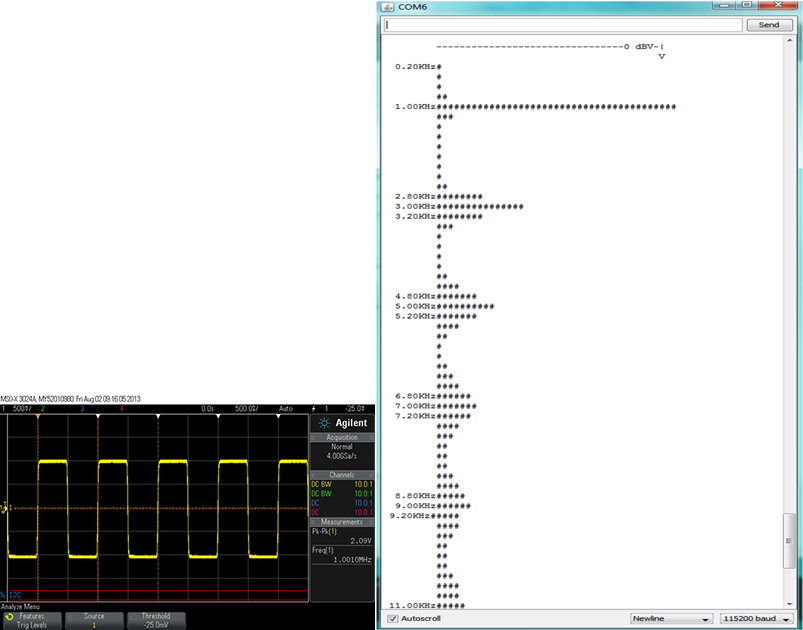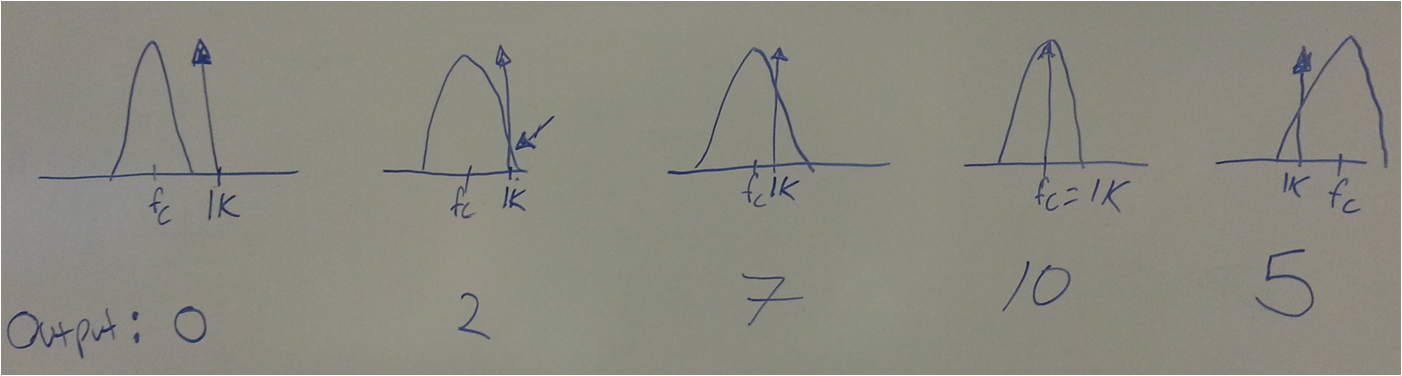# Audio Spectrum Analyzer with the Linduino

There are several methods for implementing a spectrum analyzer. I’ve been working with SAR ADC products for some time, and using the Fast Fourier Transform (FFT) for spectral analysis on a daily basis. Another common method for implementing a spectrum analyzer is called the swept-tuned receiver. This method involves mixing the input signal with a local oscillator, passing the output through an intermediate frequency filter, then finally a detector. The local oscillator is swept such that the detector’s output sweeps across the bands of interest. This blog, on the other hand, implements a spectrum analyzer using different analog techniques. To prove the concept, I used readily available hardware. This also showcases several very useful building blocks that can be incorporated into customer designs.

The technique that I used involves the LTC1068 programmable filter, configured as a bandpass, being swept across the frequencies of interest. An LTC1967 RMS to DC converter converts the filtered output to DC, and finally, an LTC2484 Delta Sigma ADC measures the voltage. Figure 1 shows the block diagram for the analyzer. Unlike the swept-tuned receiver analyzer, the filter’s center frequency is swept across the spectrum.

After a quick run to the samples department, a few demo boards were soldered to a copper clad in typical LT style (Figure 2).

A few lines of code and a fixed solder joint later, a sine wave was ready to be fed into the system. Figure 3 shows a 1kHz sine wave and the output of the Linduino. The graph is displayed sideways since a text interface was a fast and simple solution (KISS). The analyzer was set to 500 Hz steps and the filter was swept from 500Hz to 20kHz.

After having a little celebration in the privacy of my cube, I wanted to see a more interesting picture. I thought back to my Signals and Systems class from college and tried to recall the Fourier lectures. A square wave with exactly 50% duty cycle should have only odd harmonics. After tracking down a proper generator, I was armed and dangerous. Figure 4 shows the successful square wave test. The spreading around the peaks is not caused by the generator. It is the shape of the filter’s passband as it slides across the spectrum. This is analogous to the resolution bandwidth of a swept-tuned spectrum analyzer. Figure 5 illustrates the spreading and Figure 6 shows this effect on an Agilent 89410A spectrum analyzer.Figure 4. 1kHz Square Wave at 50% Duty CycleFigure 5. Filter Sideband AttenuationFigure 6. Spectrum Analyzer with Different Bandwidth. 1 Hz Bandwidth (Left). 10 Hz Bandwidth (Middle). 30 Hz Bandwidth (Right).

One aspect with this design is that the LTC1068 has constant Q factor, rather than a constant bandwidth. When the frequency increases, the bandwidth increases proportionately. The bandwidth appears constant when plotted on a logarithmic axis.

Next, let's get an accurate picture of the filter shape. To do this, a 10kHz sine wave was applied to the input and small steps were set. Figure 7 shows the hardware measured filter shape and LTspice simulated filter shape. They both used the same method for plotting the shape. In the case for LTspice, the .measure command was used to store the RMS output values to the .log file as the clock frequency was swept.Figure 7. Measured Filter Shape (Left). Simulated LTSpice Filter Shape (Right).

After some more fun, more plots were produced. Figure 8 shows more inputs. The square wave with non 50% duty cycle has odd and even harmonics and a triangle wave. Lastly, notice how that the harmonics drop off much sooner for the triangle wave than the square wave.Figure 8. 500 Hz Square Wave %20 Duty Cycle (Left) and 500 Hz Triangle Wave(Right).

The Linduino code for this project is available in the user contributed folder in the Linduino libraries at http://www.analog.com/en/design-center/evaluation-hardware-and-software/linduino